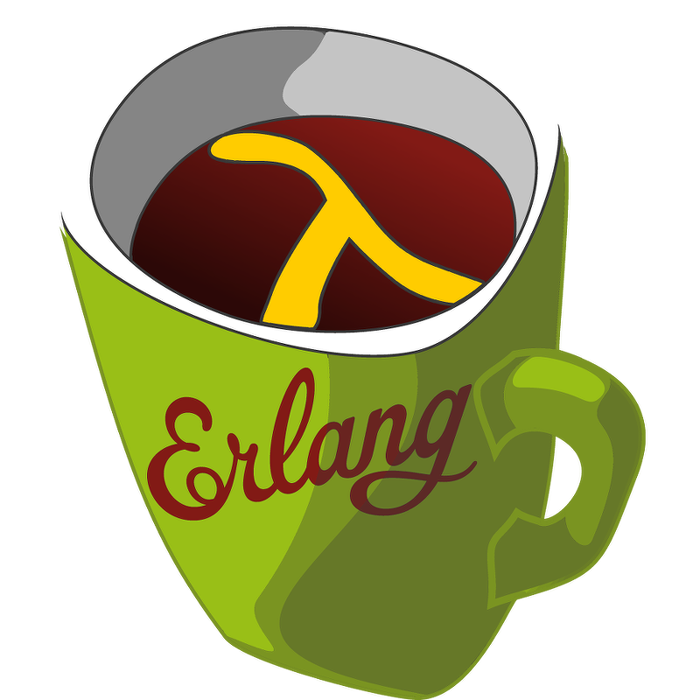This week's LFE Friday was translated with permission from the Erlang Thursday series by Steven Proctor. This week's translator: Robert Virding.

Today's LFE Friday is on digraph:del_path/3.

We will continue working with the same graph we started with in the previous post on digraph:get_path/3.

``````> (set graph (digraph:new))
#(digraph 8207 12304 16401 true)
> (set v-1 (digraph:add_vertex graph 'v-1))
v-1
> (set v-2 (digraph:add_vertex graph 'v-2))
v-2
> (set v-3 (digraph:add_vertex graph 'v-3))
v-3
> (set v-4 (digraph:add_vertex graph 'v-4))
v-4
> (set e-1 (digraph:add_edge graph v-1 v-2))
(\$e . 0)
> (set e-2 (digraph:add_edge graph v-2 v-3))
(\$e . 1)
> (set e-3 (digraph:add_edge graph v-3 v-4))
(\$e . 2)
> (set e-4 (digraph:add_edge graph v-2 v-4))
(\$e . 3)
>(set e-5 (digraph:add_edge graph v-4 v-1))
(\$e . 4)``````

`digraph:del_path/3` takes three arguments, a graph, a source vertex, and a destination vertex, and removes all edges in a each path in the graph from the source vertex to the destination vertex, until no path exist between the source and destination vertices.

The return value of `digraph:del_path/3` is always a return value of `true`.

Looking at the picture of the graph above as reference, we are going to call `digraph:del_path/3` for the graph with a source vertex of `v-1`, and a destination vertex of `v-4`.

``````> (digraph:del_path graph v-1 v-4)
true
> (digraph:vertices graph)
(v-4 v-3 v-2 v-1)
> (digraph:edges graph)
((\$e . 4) (\$e . 2) (\$e . 1))``````

Translating the edge names, we see that the edge from `v-1` to `v-2` has been removed, as well as the edge from `v-2` to `v-4` has been removed.

So how did `digraph` come up with this result?

This puzzled me at first, as it wasn't one of the two scenarios I was expecting to see, which were either: remove all edges but the edge from `v-4` to `v-1`, or remove only the edge from `v-1` to `v-2`.

I then opened the Erlang source code on GitHub for the digraph module to clarify my thinking, and looking at the code it then made sense what was happening.

First `digraph:del_path/3` calls `digraph:get_path/3`, and removes all edges in that path, and then recurses until no path is found.

This is when it clicked as to why LFE/Erlang was removing only those edges.

If we call `digraph:get_path/3` on a fresh version of the graph, we see that it returns the path of `v-1 -> v-2 -> v-4`.

``````> (digraph:get_path graph v-1 v-4)
(v-1 v-2 v-4)``````

LFE then removes the edges in that path, and the will call `digraph:del_path/3`, which calls `digraph:get_path/3` again, but as we removed the edge between `v-1` and `v-2`, no path is found so the process is finished.

This is why we see more edges removed if we reset the Graph again (by exiting the shell and recreating it from scratch by pasting the initialization into the shell), and call `digraph:del_path/3` between `v-2` and `v-4`.

``````> (digraph:del_path graph v-2 v-4)
true
> (digraph:edges graph)
((\$e . 4) (\$e . 0))``````

This case there are the paths `v-2 -> v-4` and `v-2 -> v-3 -> v-4`, and if we remove the path `v-2 -> v-4`, the removal of all the edges associated with that path doesn't break the path of `v-2 -> v-3 -> v-4`, so it can remove all edges in that path as well.

So we have a win in the case where the documentation wasn't quite as clear as it could be, but having the Erlang standard library be open source gets us a win because we can always go in and check out what the code is really doing.

- Proctor, Robert

27 November 2015

tutorials